[BACK]
 Intelligent Automation & Soft ComputingDOI:10.32604/iasc.2023.022631Article

Speckle Noise Suppression in Ultrasound Images Using Modular Neural Networks

Department of CSE, Infant Jesus College of Engineering, Anna University, Tuticorin, 628251, India
*Corresponding Author: G. Karthiha. Email: karthigapapers@gmail.com
Received: 13 August 2021; Accepted: 27 October 2021

Abstract: In spite of the advancement in computerized imaging, many image modalities produce images with commotion influencing both the visual quality and upsetting quantitative image analysis. In this way, the research in the zone of image denoising is very dynamic. Among an extraordinary assortment of image restoration and denoising techniques the neural network system-based noise suppression is a basic and productive methodology. In this paper, Bilateral Filter (BF) based Modular Neural Networks (MNN) has been utilized for speckle noise suppression in the ultrasound image. Initial step the BF filter is used to filter the input image. From the output of BF, statistical features such as mean, standard deviation, median and kurtosis have been extracted and these features are used to train the MNN. Then, the filtered images from the BF are again denoised using MNN. The ultrasound dataset from the Kaggle site is used for the training and testing process. The simulation outcomes demonstrate that the BF-MNN filtering method performs better for the multiplicative noise concealment in UltraSound (US) images. From the simulation results, it has been observed that BF-MNN performs better than the existing techniques in terms of peak signal to noise ratio (34.89), Structural Similarity Index (0.89) and Edge Preservation Index (0.67).

Keywords: Speckle noise; bilateral filter; ultra-sound image; MNN; kurtosis

1  Introduction

The US image is created by the reflection of waves of body structures. It may be a sound wave with frequencies higher than the upper capable of being heard restrain human hearing. Ultrasound devices work with frequencies from 20 kHz to a few giga hertz . US images are affected with speckle noise (SN) while procurement, transmission and retrieval process. Clatter, in US-images could be categorized as, salt and pepper, Gaussian, and SN noise. Speckle could be a specific type of noise that happens in US images gotten by articulate imaging schemes like US . It is induced by obstructions amid similar waves that, reflected from common exteriors, attain out of the stage in the sensor . Speckle noise is a common feature of images from Ultrasound. Backscattered echo signals cause speckle noise in medical US images. Because of the granular pattern that emerges in the images, speckle noise exhibits the properties of multiplicative noise and Rayleigh distribution, decreasing image resolution and contrast. Skewering noise in medical images in the US inhibits physicians from accurately diagnosing lesions as they prevent extraction, analysis and detection of lesion characteristics. A speckle noise suppression algorithm is an important pre-processing approach for obtaining an accurate lesion identification and analysis using US imaging.

A neural network (NN) method is considered to categorize SN into three wide measurable groups, and it has been utilized effectively both in likelihood thickness estimation and for filtering of multiplicative noise in US images. It is also expected that the dissemination could be described by constraints. Different characteristics are calculated from whole speckle noisy images and are in this way utilized as input to multilayer neural systems with ceaseless output values. The systems recognize parameters in two covering stages: (1) Evaluation of the parameter of speckle noise and (2) Removal of speckle noise. NN has a long history of victory in classification issues, counting infection descriptions in ultrasound scans. The system adopts Bilateral Filter (BF) based Modular Neural Networks (MNN) to suppress the speckle noise produced in the Ultrasound images. The Bilateral filter is a non-linear edge-preserving filter that reduces the noise and smoothens the input image. It smoothens the features of the image without affecting its edge. The Modified neural network system determines the intensity of features and classifies the input image. It helps to overcome the shortcomings such as fine detail loss and edge loss. It has been appeared to be all-inclusive approximations, given an adequate number of units within the networks covered up layers.

The remaining part of this work is arranged in the following way. A transitory literature review on speckle suppression techniques is presented in Section 2. The proposed methodology using BF-based MNN speckle suppression is defined in Section 3. Section 4 illustrates the simulation result and discussion. The efficiency of this method is evaluated in Section 5 and the conclusion of this paper is presented in Section 6.

2  Literature Review

SN reduction technique has been developed for 2-D US imaging based on an artificial neural network. This method can suppress the blur and speckle noise correctly . Fast removal of SN in real US images has been described by combining Adaptive filtering and Bergman iterative technique. SN reduction technique compared with the other similar speckle reduction methods gave quality results in terms of speed and speckle reduction performance . For Speckle Noise Reduction and the Boundary Enhancement on Medical US Images, Cellular Neural Networks (CNN) has been proposed . Neural Network using the IIR (Infinite Impulse Response) filter (IIRNN) technique was used in the learning stage. This technique was efficiently removed the speckle noise in US images .

Even though the NN is active in noise suppressing systems, it is suffered from the intricacy of computations and the length adjustment of the parameters of neural systems. Although all of the outputs’ pixel values are scaled to span the whole gray scale range, most existing methods fail to recover contrast and structural features.

This confines the handy utilization of such versatile systems to a stationary or partially stationary noise source. Also, the NN is over-trained for the identification of speckle noise. These above-mentioned drawbacks of NN are overcome by utilizing the BF-based MNN speckle suppression systems.

3  Proposed Method

The block diagram of proposed speckle suppression using BF and MNN is given in Fig. 1. In this method, two stages of the de-speckling process have been performed. During the first stage, bilateral filter has been used for the filtering process. Then, the denoised image and the original ultrasound images are subtracted to get the filtered speckle noise. After that, the statistical characteristics such that mean, median, standard deviation and kurtosis are calculated from the filtered speckle-noise image. These features are utilized to train MNN. In the second stage of the filtering process, these trained MNN has been used to de-speckle the filtered image which is from the output of the bilateral filter. At last, the performance metrics such that, Peak signal to noise ratio (PSNR), Structural Similarity Index (SSIM) and Edge Preservation Index (EPI) are evaluated to get the efficiency of the BF-MNN filtering method.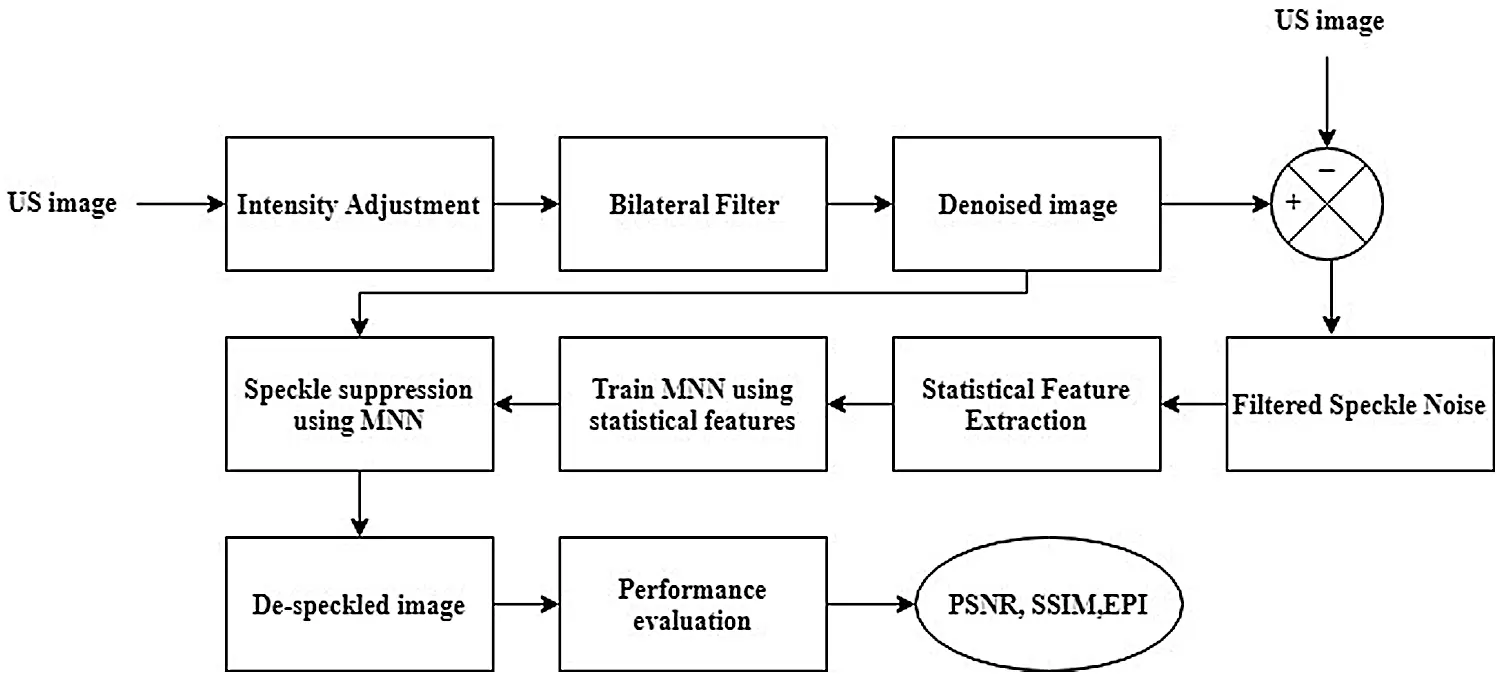Figure 1: Block diagram of speckle suppression using BF based MNN

Initially, the US image is given as input to the intensity adjustment block as shown in Fig. 1. Here, Histogram Equalization (HE) method has been used for intensity adjustment. HE is performed by remapping the dim degrees of an image dependent on the likelihood dissemination of the input gray levels. It straightens and extends the dynamic scope of the image histogram and bringing in an overall contrast enhancement image.

3.2 Bilateral Filter

Bilateral filter can convert any form of input image into smooth noiseless image without deconstructing the sharp edge surface. The intensity esteems at every pixel in a denoised image are supplanted by an average of pixel intensity esteems from neighboring pixels. This weight can be founded on Gaussian distribution. Vitally the weight does not only depend on Euclidean separation but depends on the radiometric distinction. These characteristics of bilateral filter preserve the sharp edges by efficiently circling through every pixel and modifying loads to the contiguous pixels accordingly. All of the images are 400 × 400 pixels in size. Each image was denoised using a Bilateral filter with varying levels of thresholds. The objective function of bilateral function is expressed as,

BFiltered=xiDW(xikr(DW(xi)DW(x))ks(xix)) (1)

where BFiltered is the de-speckled image, DW is the filtered image using soft thresholding with SURE estimation, x is the coordinates of the current pixel to be filtered, kr is the kernel range and ks is the spatial kernel function for smoothing images.

3.3 Statistical Feature Extraction

Feature extraction is the method of changing over an input image into a set of characters or properties of an image. It was finding the set of images that describe the shape of a character absolutely and interestingly. The main objective of feature extraction is to extract a set of characters, which maximizes the identification rate with the slightest number of components and to produce a comparable feature set for several instances of the same image. Many image attributes can be utilized to train the Modular Neural Network. In this system, the first-order statistics attributions like mean ( μ ), standard deviation (σ) , kurtosis (k) and median (m) are calculated to extract the set of characters. The mathematical expression to derive the statistical attribution is given below;

μ=1PQIPJQfi,j (2)

σ=1PQI=1P.J=1Q(fi,jμ)2 (3)

k=PQi=1Pj=1Q(fi.jμ)4(i=1Pj=1Q(fi,jμ)2)2 (4)

Here PQ indicates the number of values, fi,j indicates the data set values.

m=sm+sm+12 (5)

Here sm indicates the number of sample observations.

3.4 Speckle Suppression Using MNN

The Neural Network (NN) design comprises three layers namely, input, intermediate and output layer. This system and its associations have appeared in Fig. 2. Every unit of input and the intermediate layer are organized into 2 measurements. One size of information is a standardized estimation of the intensity of an image. The highest value is taken as 1 and the lowest value is taken as –1. The input layer is taken for processing by reading the data to fit in the layer size for the corresponding input image. Each unit in the input layer of the non-linear function yields the resultant information to the hidden layer. By adding weight addition to the input data, the results are generated for the hidden and output layer. This procedure continues moving a position a pixel through the image and is appeared in Fig. 2. Each intensity value of the input image is compared with the output of NN. To minimize the error the internal conditions are altered. The back propagation algorithm is used to fully train the NN and MNN system is shown in Fig. 2. Each layer that is used in MNN is briefly explained underneath and the modular neural network is shown in Fig. 3.Figure 2: Speckle noise suppression using MNN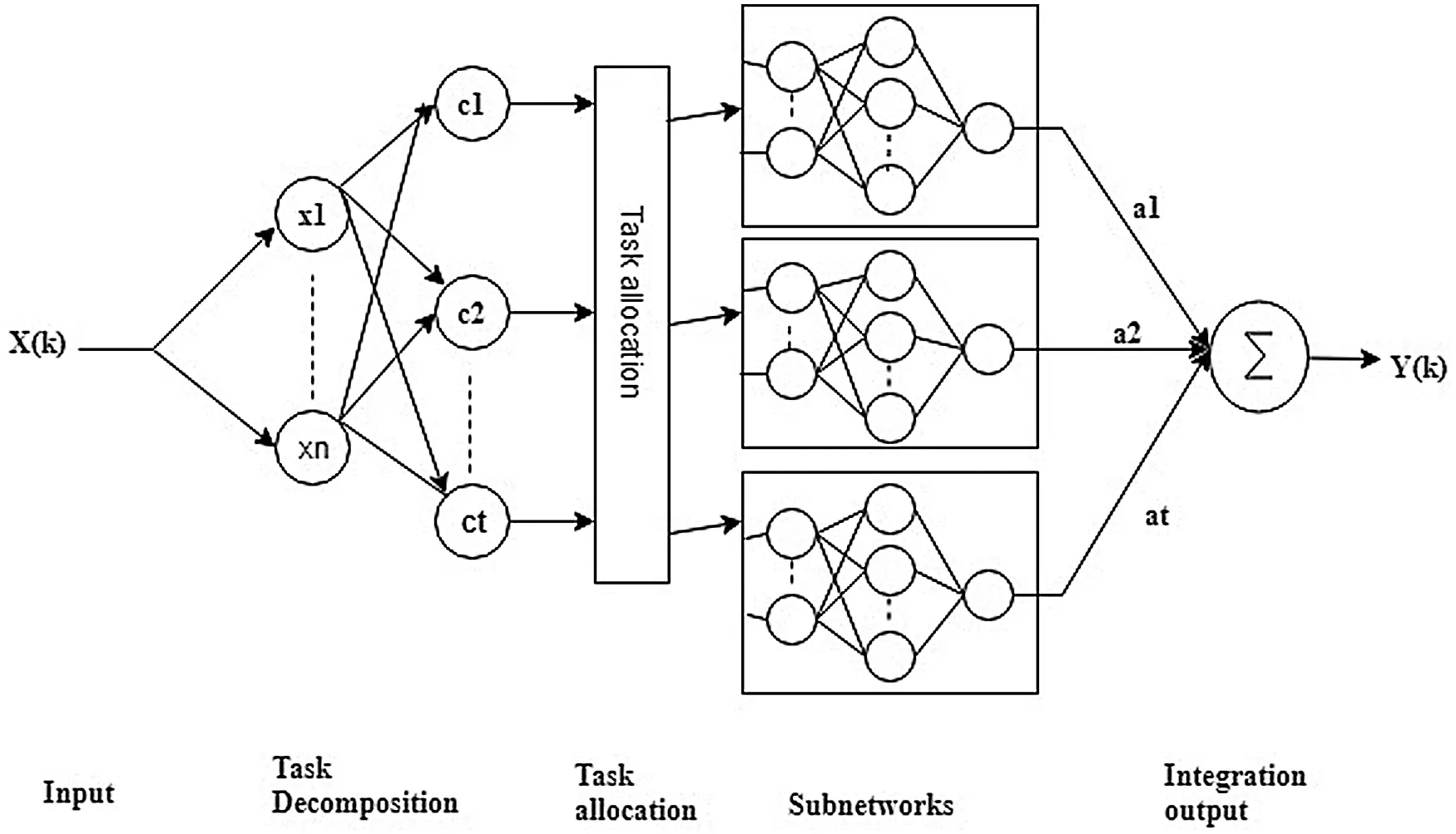Figure 3: Modular neural network (MNN)

3.4.1 Input Layer

The initial layer of MNN system is input layer. It comprises of ‘n’ basis hubs, where ‘n’ - information factor. The input ultrasound image is sectioned into n number of kernels and it is calculated as follows,

x(k)=[x1(k),x2(k)..,xn(K)]T (6)

K - Kernels in the input layer.

Prior to training the modules, task decomposition is performed using a clustering technique. It comprises a single neuron, and every neurocyte includes a kernel function which is gathered as follows,

kh(x(k),ch)={1,|x(k)ch|δh;0,|x(k)ch|δh; (7)

where, δh—ambit of the hth neurocyte.

This layer accepts the methodology that, incase kh(x(k),ch)=1 , the hth sub network made active to understand the input trial. The input trials help to powerfully balance the active sub-network.

3.4.4 Sub Network Layer (SL)

The subsampling layer or pooling layer frequently follows a Task allocation layer. Its function is to down sample the output of a AL layer along both the spatial dimensions of height and length. The feature maps’ dimensions are reduced using Pooling layers. It lowers the number of parameters that need to be learned and the calculation that is done in the network. The output is denoted in the sub network layer as follows,

yh(k)=i.=1mwi(k)f[i=1nvii(k)xi(k)] (8)

where ,wi - weights of the intermediate and output neurocyte vii - weight of input and intermediate neurocyte in SL,

f(x)=1(1+ex) (9)

3.4.5 Integration Output Layer

The output of the MNN network is calculated in the integration output layer as follows,

Y(k)=g=1Rαg(k)yh(k) (10)

where, ‘R’ represents no. of certain sub networks 1Rl,αg(k) is the integration weight.

The architecture of the modular neural network (MNN) is shown in Fig. 3. The algorithm of the MNN system involves four main processing layers and each layer has different functional steps;

Step 1: Initially, the input ultrasound image is sectioned into the ‘n’ number of kernels and a constant bias is added for further processing.

Step 2: The next step is task compression and Task Allocation Layer for neuron selection and feature extraction.

Step 3: Next step is sub network layer, it lowers the number of parameters that need to be learned and the calculation that is done in the network. It remove unwanted speckle noise in multiple process.

Step 4: The Final step is Integration Output Layer. It concatenates the feature of sub networks and produce the output.

Both Bilateral filter and Modular neural network (MNN) produce effective results over the filtering and detection of speckle noise in any form of ultrasound image. The BF filter removes the noise and texture without affecting the sharp edges and smoothen the image.

4  Simulation Results and Discussion

US dataset from the Kaggle site has been used for this experiment. It consists of two groups namely benign and malignant. Benign consists of 100 images and malignant contain 150 images. Therefore, this US dataset contains 250 ultrasound images that are affected by speckle noise. In this experiment, SN is not additionally added, because the images themselves contain SN. These images are directly used for the SN reduction process.

The statistical features of speckle-noise  such as mean, median, standard deviation and Kurtosis have been evaluated from the filtered speckle noisy image. These features are used to train the MNN, and it is trained by using the back propagation technique. This network is trained multiple times for each image. The training is continued till the system reaches the minimum error of 0.074. The parameters used for training the network and the error after the training are presented in Tab. 1.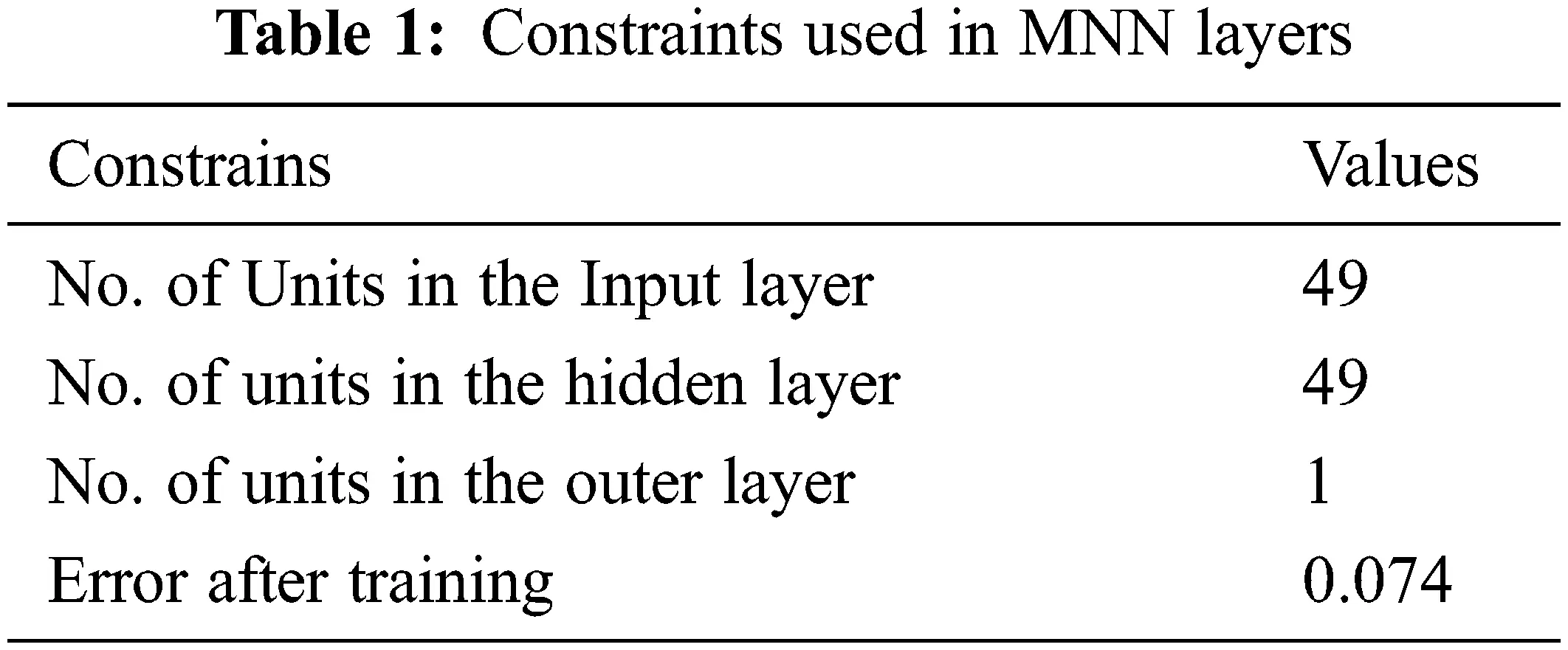The simulation results of US benign image using BF-MNN method and the various existing methods such as DTCWT (Dual Tree Complex Wavelet Transform), IIR and Nl are given in Figs. 4 and 5. Similarly, experimental outcomes of US malignant image using BT-MNN, DTCWT, IIR and NL are given in Figs. 6 and 7.Figure 4: Simulation outcomes of BF-MNN and various existing de-noising methods for benign-01 US image. (a) Input US image, (b) BF-MNN, (c) DTCWT, (d) IIR, (e) NL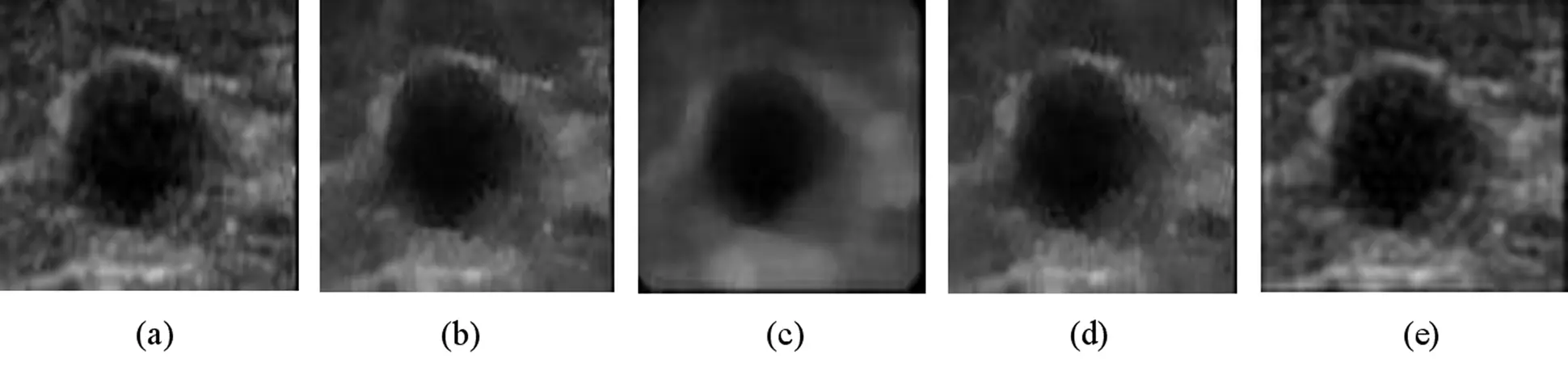Figure 5: Simulation results of BF-MNN and various existing de-noising methods for benign 02 US image. (a) Input US image, (b) BF-MNN, (c) DTCWT, (d) IIR, (e) NL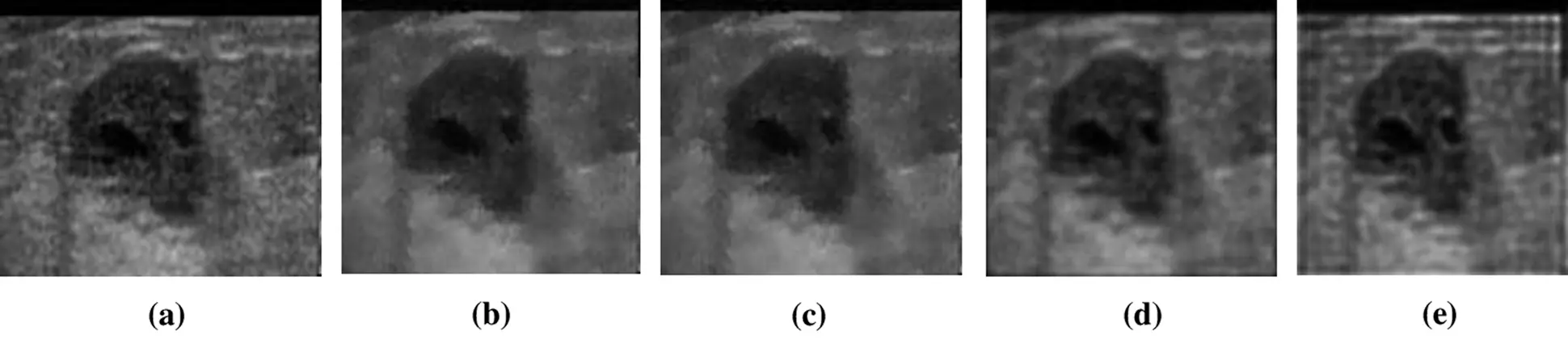Figure 6: Experimental outcomes of BF-MNN and various existing de-noising methods for malignant-01 US image. (a) input US image, (b) BF-MNN, (c) DTCWT, (d) IIR, (e) NLFigure 7: Experimental outcomes of BF-MNN and various existing de-noising methods for malignant-02 US image (a) input US image (b) BF-MNN (c) DTCWT (d) IIR (e) NL

Figs. 47 shows the experimental outcome of both benign and malignant ultrasound medical images. Here the outcome of proposed BF-MNN technique is compared with existing techniques such as DTCWT, IIR & NL. From the simulation results of benign and malignant US images, it is observed that the DTCWT method produced good speckle-noise suppressed images. In this method, some of the useful information is also removed from the original image, and the edges are not preserved properly. In IIR, some of the important attributes of original images are removed from the image. Speckle suppression using NNL produces poor segmentation results when compared with other methods. In this method, the speckle noise is not filtered properly comp. After visually notifying all the simulation results, the proposed method BF-MNN produced a better quality of filtered images.

5  Performance Evaluation

Performance of hybrid de-speckling method based on MNN and improved bilateral filter can be assessed utilizing numerical proportions of image quality after the filtering method has been performed on the image. The presentation measurements are selected dependent on their processable contortion measures. Peak signal-to-noise ratio (PSNR) and Mean square error (MSE) are the two most well-known proportions of image quality in image processing systems [19,20]. In addition to these parameters, EPI (Edge Preservation Index) and SSIM (Structural Similarity Index Metric) have also been evaluated to find the efficiency of the hybrid de-speckling method. The PSNR and the MSE measures are given by Eqs. (11) and (12), respectively,

PSNR=10log(255)2MSE (11)

MSE=m=0P1n=0Q1(x(m,n)y(m,n))2P×Q (12)

The SSIM metric can be described as,

SSIM(x,y)=(2μxμy+C1)(2σxy+C2)(μx2+μy2+C1)(σx2+σy2+C2) (13)

Another parameter EPI can be calculated by using the equation,

EPI=(ΔxΔx¯)(ΔyΔy¯)(ΔxΔx¯)2(ΔyΔy¯)2 (14)

where, x(m, n) - pixel esteems in the noise-free US-image, y(m, n) - pixel esteems in the de-speckled image after the filtering process, P, Q is the size of the image, and µx, µy, σx, σy, and σxy are the local means, standard deviations and cross-variance respectively.

In this paper, around 30 US images have been simulated and the values are plotted in Fig. 8. The average values of PSNR, SSIM, and EPI are calculated by using those values and tabled in Tab. 2. From this tabulation, it can be noticed, the proposed methodology BF-MNN method produces better results in terms of PSNR of 34.89, SSIM of 0.89 and EPI of 0.67. This method is compared with DTCWT-IBTV, IIR and NL methods. Here, the DTCWT method produces the output filtered images with PSNR of 34.36, SSIM of 0.89 and EPI of 0.65. The IIR produced de-speckled images with the PSNR of 33.83, SSIM of 0.84 and EPI of 0.62. At last, the NL produces the denoised images with the PSNR of 32.85, SSIM of 0.72 and EPI of 0.59.Figure 8: Graphical representation of values of performance metrics for various US images. (a) PSNR (b) SSIM and (c) EPIThe above comparison of performance metrics of various exiting methods is graphically represented in Fig. 9. The proposed method BF-MNN method shows improvements in terms of PSNR, SSIM and EPI over other various de-speckling methods. Therefore, the BF-MNN method is well suitable for de-speckling the various US images.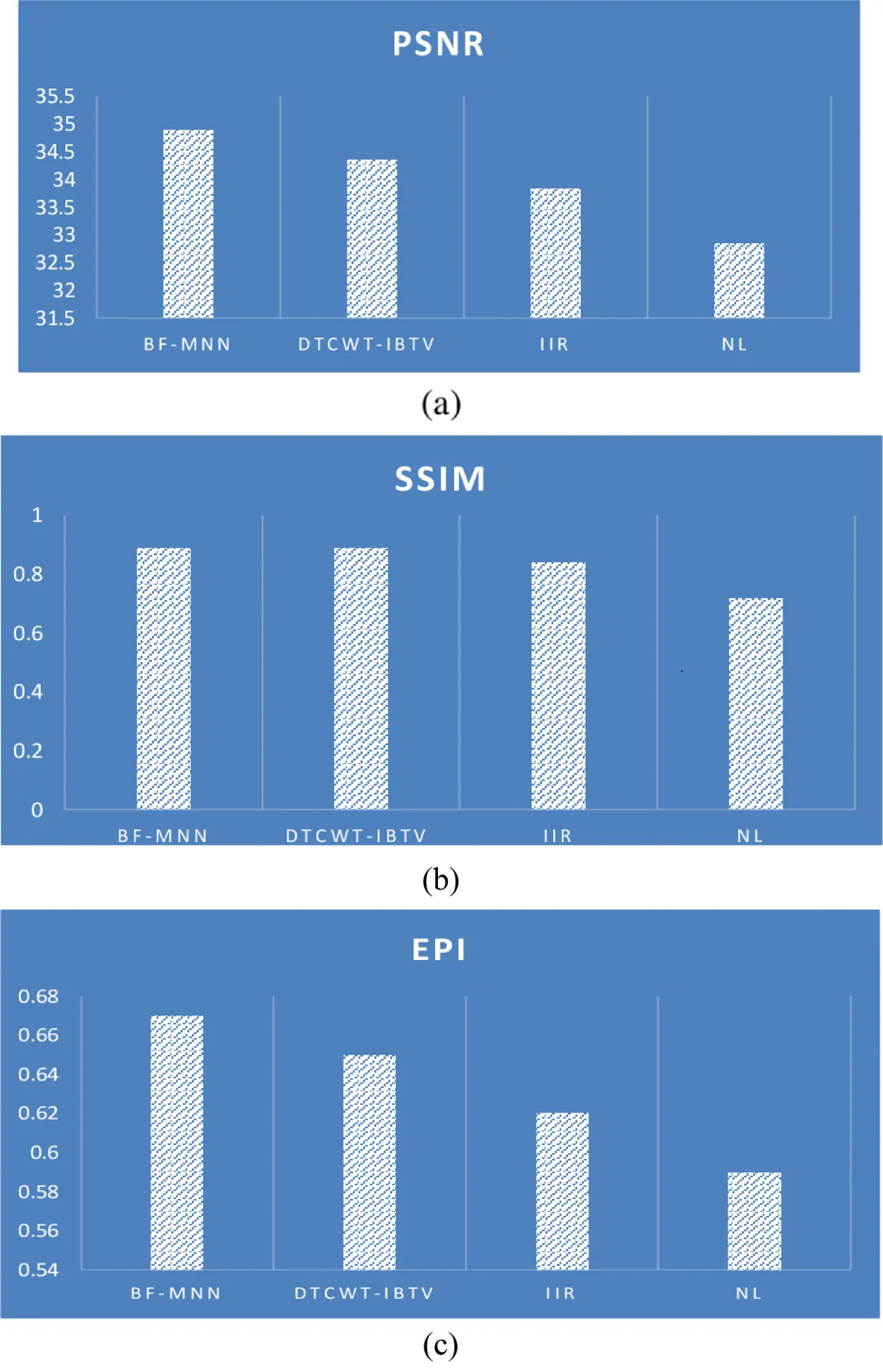Figure 9: Comparison of performance metrics of various exiting methods. (a) PSNR (b) SSIM (c) EPI

Fig. 9 shows the performance metrics comparison of proposed BF-MNN technique with the existing speckle noise depression techniques such as DTCWT, IIR and NL. The proposed method BF-MNN shows improvements in terms of PSNR, SSIM and EPI over other various de-speckling methods. Therefore, the BF-MNN method is well suitable for de-speckling the various US images.

6  Conclusion

This paper has concentrated on ultrasound images more explicitly, on the concealment techniques for the multiplicative noise. This work has contrasted with various denoising techniques used to suppress the SN in medicinal images acquired through ultrasound images. BF-MNN filtering method has been utilized to suppress the SN in US images. The simulation outcomes demonstrate that the BF-MNN filtering method performs better for the multiplicative noise concealment in US images. The proposed method BF-MNN method shows improvements in terms of PSNR, SSIM and EPI over other various de-speckling methods such as DTCWT-IBTV, IIR and NL methods. The better quality of denoised images is observed by using BF-MNN over other methods such that DTCWT-IBTV, IIR and NL. The proposed method BF-MNN produced a better quality of de-speckled images with the PSNR of 34.89, SSIM of 0.89 and EPI of 0.67 values for US images.

Acknowledgement: The authors would like to thank Anna University and also, we like to thank anonymous reviewers for their so-called insights.

Funding Statement: The authors received no specific funding for this study.

Conflicts of Interest: The authors declare that they have no conflicts of interest to report regarding the present study.

## References

1. P. Kalavathi, S. Boopathiraja and M. Abinaya, “Despeckling of ultrasound medical images using DW and WP transform techniques,” International Journal of Engineering and Technology, vol. 9, no. 3, pp. 123–130, 2017.
2. P. Singh and L. Jain, “Noise reduction in ultrasound images using wavelet and spatial filtering techniques,” in Proc. Int. Conf. on Information Management in the Knowledge Economy, Chandigarh, India, IEEE, pp. 57–63, 2013.
3. L. Gagnonand and A. Jouan, “Speckle filtering of SAR images: A comparative study between complex-wavelet-based and standard filters,” Wavelet Applications in Signal and Image Processing V, vol. 3169, pp. 80–91, 1997.
4. H. Park and T. Nishimura, “Reduced speckle noise on medical ultrasound images using cellular neural network,” in Proc. Int. Conf. of the IEEE Engineering in Medicine and Biology Society, Lyon, France, IEEE, pp. 2138–2141, 2007.
5. S. Shawal, M. Shoyab and S. Begum, “Fundamentals of digital image processing and basic concept of classification,” International Journal of Chemical and Process Engineering Research, vol. 1, no. 6, pp. 98–108, 2014.
6. A. Gupta, V. Bhateja, A. Srivastava, A. Gupta and S. C. Satapathy, “Speckle noise suppression in ultrasound images by using an improved non-local mean filter,” in Proc. Soft Computing and Signal Processing, Singapore, Springer, pp. 13–19, 2019.
7. N. Bender, H. Yılmaz, Y. Bromberg and H. Cao, “Customizing speckle intensity statistics,” Optica, vol. 5, no. 5, pp. 595–600, 2018.
8. Y. Xu, J. B. Weaver, D. M. Healy and J. Lu, “Wavelet transform domain filters: A spatially selective noise filtration technique,” IEEE Transactions on Image Processing, vol. 3, no. 6, pp. 747–758, 1994.
9. H. Choi and J. Jeong, “Speckle noise reduction in ultrasound images using SRAD and guided filter,” in Proc. Int. Workshop on Advanced Image Technology, Chiang Mai, Thailand, IEEE, pp. 1–4, 2018.
10. B. H. S. Asli, J. Flusser, Y. Zhao, J. A. Erkoyuncu, K. B. Krishnan et al., “Ultrasound image filtering and reconstruction using DCT/IDCT filter structure,” IEEE Access, vol. 8, pp. 141342–141357, 2020.
11. D. Feng, W. Wu, H. Li and Q. Li, “Speckle noise removal in ultrasound images using a deep convolutional neural network and a specially designed loss function,” in Proc. Multiscale Multimodal Medical Imaging, Lyon, France, Springer, pp. 85–92, 2019.
12. P. Coupe, P. Hellier, C. Kervrann and C. Barillot, “Bayesian non-local means-based speckle filtering,” in Proc. Biomedical Imaging: From Nano to Macro, Paris, France, IEEE, pp. 1291–1294, 2008.
13. M. Badawi and M. A. Rushdi, “Speckle reduction in medical ultrasound: A novel scatter density weighted nonlinear diffusion algorithm implemented as a neural-network filter,” in Proc. Int. Conf. of the IEEE Engineering in Medicine and Biology Society, New York, NY, USA, IEEE, pp. 2776–2782, 2006.
14. M. S. Uddin, K. K. Halder, M. Tahtali, A. J. Lambert and M. R. Pickering, “Speckle reduction and deblurring of ultrasound images using artificial neural network,” in Proc. Picture Coding Sym., Carins, QLD, Australia, IEEE, pp. 105–108, 2015.
15. J. Huang and X. Yang, “Fast reduction of speckle noise in real ultrasound images,” Signal Processing, vol. 93, no. 4, pp. 684–694, 2013.
16. H. Park, R. Miyazaki, T. Nishimura and Y. Tamaki, “The speckle noise reduction and the boundary enhancement on medical ultrasound images using the cellular neural networks,” IEEJ Transactions on Electronics, Information and Systems, vol. 127, no. 10, pp. 1726–1731, 2007.
17. M. Ranjitha, “Extraction and dimensionality reduction of features for renal calculi detection and artifact differentiation from segmented ultrasound kidney images,” in Proc. Int. Conf. on Computing for Sustainable Global Development, New Delhi, India, IEEE, pp. 3087–3092, 2016.
18. O. Karaoglu, H. S. Bilge and I. Uluer, “Removal of speckle noises from ultrasound images using five different deep learning networks,” Engineering Science and Technology, an International Journal, vol. 3, no. 5, pp. 13–19, 2021.
19. M. Lal, L. Kaur and S. Gupta, “Speckle reduction with edge preservation in B-scan breast ultrasound images,” International Journal of Image, Graphics and Signal Processing, vol. 8, no. 9, pp. 60–68, 2016.
20. M. S. Uddin, M. Tahtali, A. J. Lambert, M. R. Pickering, M. Marchese et al., “Speckle-reduction algorithm for ultrasound images in complex wavelet domain using genetic algorithm-based mixture model,” Applied Optics, vol. 55, no. 15, pp. 4024–4035, 2016.This work is licensed under a Creative Commons Attribution 4.0 International License, which permits unrestricted use, distribution, and reproduction in any medium, provided the original work is properly cited.